# Implementing a BST where every node stores the maximum number of nodes in the path till any leaf

## Introduction

Let us understand the problem statement. We are given an array of integers ‘INPUT'. We need to construct a binary search tree by inserting the values from 'INPUT' sequentially. In addition to that, we need to calculate the node's height in the tree for every node. Height is defined as the maximum number of nodes in the path starting from the node itself and ending at any leaf node (excluding the node itself).

Example:

Input: INPUT = [10, 5, 2, 6, 7], the values in the input array must be unique.

Output:

ANS =

[

[2, 0],

[5, 2],

[6, 1],

[7, 0],

[10, 3]

]

Explanation: The output is a 2D array. The first element in every row corresponds to a node's value and the second element corresponds to the node's height in the tree. Moreover, if you observe closely, you will find that the nodes are traversed in an inorder fashion while constructing the output.

Below is a series of tree diagrams that will help you visualize how insertion is happening in the tree and how heights are updated.

INSERT(10)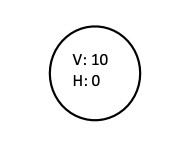INSERT(5)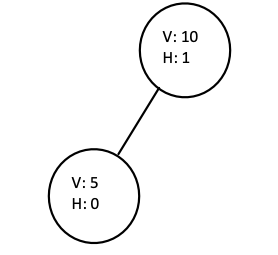INSERT(2)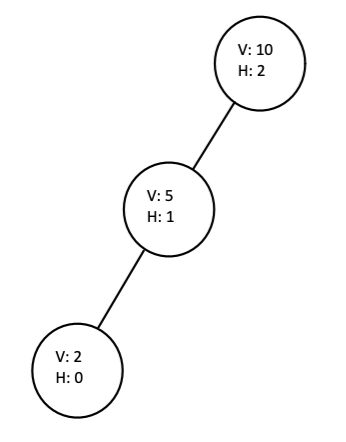INSERT(6)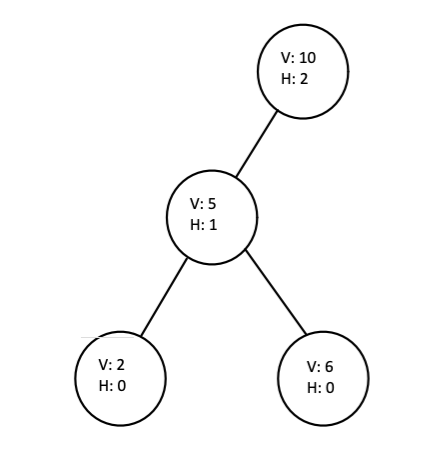INSERT(7)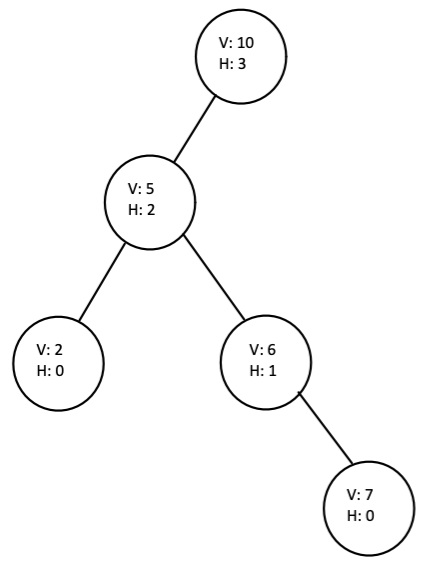Note: "V" stands for value, "H" stands for height.

## Solution Approach

To understand how insertions are taking place in the binary search tree, you first need to understand what is a binary search tree. Follow the link above to get a basic understanding of binary search trees.

• First, we will define our own data structure, "TreeNode". It will have five fields:
• VALUE: stores the value of the node.
• HEIGHT: stores the height of the node in the binary search tree.
• LEFT: stores the reference to the left child
• RIGHT: stores the reference to the right child
• PARENT: stores the reference to the parent

This will make the code implementation a lot easier.

• Next, we will iterate through the array and insert nodes into the binary search tree. Let's say we need to insert a node with the value 'X'. We will maintain two pointers, 'PREV' and 'CURR'. CURR is pointing to the root node, and PREV is pointing to NULL.
• Every time we compare X and CURR's value until CURR is NULL. There are two cases possible,
• X < CURR's value: PREV stores the reference of CURR, and CURR is updated to its LEFT child.
• X > CURR's value: PREV stores the reference of CURR, and CURR is updated to its RIGHT child.
• When CURR is NULL, we create a new TreeNode with value 'X' and height 0. PARENT of this node is updated to store the reference of PREV. If X < PREV's value, PREV's LEFT child is updated, else PREV's RIGHT child is updated to store the reference of the newly created node. We have successfully inserted a node into the binary search tree, and CURR is made to point to this node.
• Now we need to update the heights. The height of the node inserted is 0 because it is a leaf node. We will climb up the tree using the reference of PARENT. When PARENT's height is less than CURR's height + 1, we will update the height of the PARENT and update CURR to point to its PARENT. Else we would break out of the loop.

## C++ Code

``````#include <bits/stdc++.h>
using namespace std;

// Defining the structure of TreeNode
struct TreeNode
{

/*
A TreeNode has 5 fields:
value: to store the value
of the node
height : to store the height
of the node in the tree
left : to store the reference to
the left child
right : to store the reference to
the right child
parent : to store the reference to
the parent
*/

int value, height;
TreeNode *left, *right, *parent;
TreeNode(int x)
{
this->value = x;
this->height = 0;
this->left = NULL;
this->right = NULL;
this->parent = NULL;
}
};

TreeNode *root = NULL;

/*
Function which update the heights
of the nodes upon insertion
*/

void updateHeight(TreeNode *curr)
{
while (curr->parent != NULL and curr->parent->height < curr->height + 1)
{
curr->parent->height = curr->height + 1;
curr = curr->parent;
}
}

/*
Function to insert node in
the binary search tree
*/

void insertNode(int x)
{

// Creating a new node
TreeNode *node = new TreeNode(x);

/*
When the tree is empty,
insertion of the fisrt node
*/
if (root == NULL)
{
root = node;
}

// Inserting subsequent nodes
else
{
TreeNode *curr = root;
TreeNode *prev = NULL;
while (curr != NULL)
{
prev = curr;
if (x < curr->value)
{
curr = curr->left;
}
else
{
curr = curr->right;
}
}

TreeNode *newNode = new TreeNode(x);
if (x < prev->value)
{
prev->left = newNode;
}
else
{
prev->right = newNode;
}
newNode->parent = prev;

// Updating the height of the nodes
updateHeight(newNode);
}
}

/*
Traversing the tree (inorder)
to generate the output
*/

void inorder(TreeNode *root)
{
if (root == NULL)
return;
inorder(root->left);
cout << "value: " << root->value << ", height: " << root->height << endl;
inorder(root->right);
}

int main()
{
vector<int> input{10, 5, 2, 6, 7};
for (int i = 0; i < input.size(); i++)
{
insertNode(input[i]);
}

/*
Traversing the tree
and printing the node's
value and height
*/
inorder(root);
}``````

## Complexity Analysis

Time Complexity: O(N2)

• Let the number of elements in the INPUT array be 'N'. To insert a node in the binary search tree, we have to go down the height of the tree, and in the worst case, the height is 'N' (think about a skewed tree).
• Also, to update the heights, after insertion, we need to climb up the tree till the root (in the worst case), so the overall time complexity for insertion + height update is O(N). And there are N values in the array, so the overall time complexity of the solution becomes O(N * N).

Space complexity: O(N)

Every node has five fields (which is a constant). Hence the space consumed by a single node is constant. There are N nodes. Hence the overall space complexity becomes O(N).

## FAQs

1. What are the average and the worst-case times to insert a node in the binary search tree?
Average case: Ө(H), worst case: O(N), where H is the height of the tree and N is the number of nodes in the tree.

2. What is a Binary search tree?
It is a binary tree, where all the nodes in the left subtree have a value less than the root, and all the values in the right subtree have a value greater than the root, and this is true for all the subtrees.

## Key Takeaways

In this blog, we solved an exciting problem tree problem: "Implementing a BST where every node stores the maximum number of nodes in the path till any leaf". While solving this problem, we primarily learned about binary search trees and the insertion of a node in a binary search tree with slight modification. We also analyzed the time and space complexity of the solution approach discussed.

Always try to analyze the time and space complexity of your solution because you will be asked to find it during a real interview.

Check out interview prep course, to enhance your problem-solving ability and ace interview rounds of big companies like Google, Amazon, Microsoft.